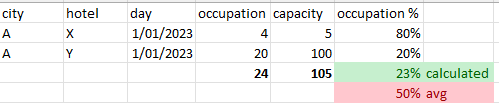# I need a division (of two numeric columns) in a model that summarizes correctly

I have a simplified sample of what I want to attain in the screenshot below:I want to add a division in my model that gives a correct result on every (grouping by) level: it needs to first summarize the numerator ('occupation'), summarize the denominator ('capacity') and then divide the summarized results. This is not the same thing as doing the division on the raw data and then somehow (avg?) summarizing it as you can see in my screenshot.

I know that in a 'question', i can add this as a custom summarization, but I really want to have this kind of calculations in my model, predefined and ready to be used by my (not so technical) end users.

Is this possible in Metabase?